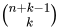COMBINA function

The COMBINA function returns the number of ways to choose some number of objects from a pool of a given size of objects, including ways to choose the same object multiple times (also known as choosing with replacement).

Parts of a COMBINA function

`COMBINA(n, k)`

 Part Description Notes `n` The size pool of objects to choose from. Given n values should be greater than or equal to 0. `k` The number of objects to choose. Given k values should be greater than or equal to 0.

Sample formulas

`COMBINA(5, 3)`

`COMBINA(A1, B1)`

Notes

• The order of the chosen objects within the COMBINA function doesn't matter.
• `COMBINA(n, k)` is equivalent to `COMBIN(n+k-1)`, which can be read as "(n+k-1)choose k" or.
• `COMBINA(n, k)` is also equivalent to `FACT(n+k-1)/(FACT(k)*FACT(n-1))`, but supports larger numbers as arguments.
• If a number (or reference to a number) with a decimal part is provided to COMBINA, the decimal part is silently truncated before calculation.
• If the some of arguments n+k is greater than or equal to 1031, a #NUM! error is returned.

Examples

In the following example with a pool of 2 objects (for example, A and B), there are 3 possible chosen combinations: (A, B), (A, A), and (B, B):

 A B 1 Formula Result 2 =COMBINA(2, 2) 3

In this example with a pool of 5 objects, there are 35 possible chosen combinations of 3 objects:

 A B 1 Formula Result 2 =COMBINA(5, 3) 35

Related functions

• FACT: The FACT function returns the factorial of a number.
• COMBIN: The COMBIN function returns the number of ways to choose some number of objects from a pool of a given size of objects.